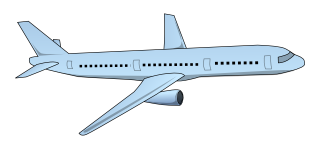My blog has been moved to ariya.ofilabs.com.

## Sunday, August 09, 2009

### how far is that planeThis did happen in my last flight. I consider making it a quick math quiz for any candidate I have to interview in the future (to see whether it takes him 5 minutes or 5 hours to analyze).

A few minutes after the captain announced that we were flying at forty thousand feet above the sea level, cruising at the speed of 900 km/h, through the window we saw another airplane flying at roughly the opposite direction, but at a slightly lower altitude. Of course, the obvious question was asked: How far is the other plane from us? This is better than the typical Fermi problem. Assuming that that airplane was visible in our 90 degrees field of view for 4 seconds and it flew just as fast as our plane, I did a quick calculation and came up with an answer, the flight trajectory of that plane was 1 km apart from ours.

The other guy was impressed, but he was not convinced. Now, since my math skills are rusty, I am sure I missed a thing or two. Now, what is your take?

(Picture rendered from Jarno's public-domain vectorized aircraft).

Dion Moult said...

I got 2km. But don't take it from me I'm not too confident in my math either :P

In fact, I think I'm completely missing the question here. All I did was relative speed = 1800kmph for 4 seconds.

I'm probably wrong.

Beat Wolf said...

i got 1km.

@Dion: what you calculated was the length of your 90degree viewfield. Which is one side of a triangle, the other thing you know is one angle (90) of that triangle, which means the two other sides are the same length.. and yeah, then you go on until you got the height of that triangle(which is the distance to the plane after 2 seconds of seeing it, the question did not specify at what point you want the distance)

Enrico Ros said...

1km for me too ((900+900)/3600*(4/2)/tan(45))

Dion Moult said...

@BeatWolf: ah yes, sorry misunderstood the question ;) Thanks!Anonymous said...

Since you estimated relative radial velocity (pi/8 rad/s): r = 2*900/3600 *8/pi = 4/pi = 1km.Anonymous said...

Why even use trig? The fact that we know that our triangle edge has an angle of 90' and the opposite side is parallel means we can easily subdivide by hand.

If both planes are travelling at 900km/h in opposite directions then the relative velocity is 1800km/h. Divide by the time taken to pass to get the length of that side of the triangle: 1800/60/60*4 = 2km

As the opposite is parallel we know we have an isosceles triangle, thus we can split the triangle equally length-wise easily to form another triangle. Splitting will get us an angle of 90'/2=45' on our edge, 90' on the far edge and 180'-90'-45'=45' on the side edge... We have another isosceles triangle! As the length of both edges on the isosceles triangle that are adjacent to the far 90' angle are the same length the distance between the two planes is 2km/2 = 1km

Diagram 'cause I suck at descriptions: http://img15.imageshack.us/img15/4717/omgplanes.pngAnonymous said...

Correction: I meant multiply by time instead of divide. A speed is distance/time so to cancel the time you need to multiply.

Of course this calculation ignores that the altitudes are slightly different. As the error would probably only be ~10% at most it can be ignored for your use case, however if you want to say shoot out one of the windows of the other plane with a sniper rifle you would need to take altitudes into account (As well as air pressure, wind, bullet velocity/spin, plane vibration and other aerodynamic stuff).

Anselmo Melo said...

I got 1 km too. 1800km/h for 4 seconds give us 2km as the hipotenuse of a triangle with the rectangle angle placed in our aircraft. The height of that triangle is the minimum distance, 1km. Of course it's only an approximate result =)Anonymous said...

for sure it's 1km..Anonymous said...